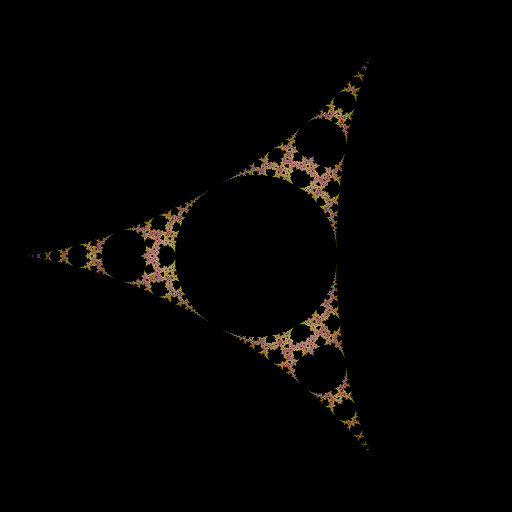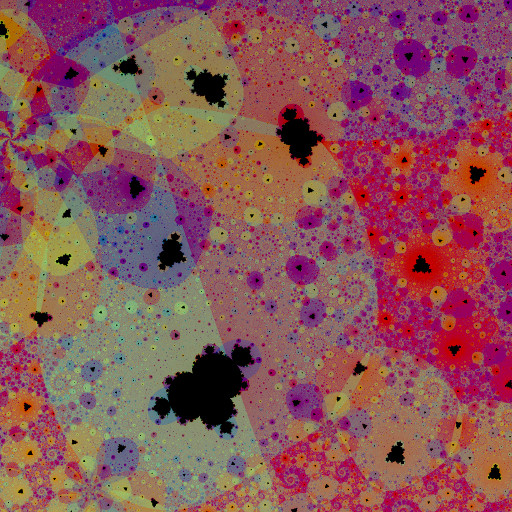# mathr / blog / #

## Lyapunov atom domains for negative multibrot setsBack at the end of April last year I think I was futzing about on math.stackexchange.com answering a question about rendering negative multibrot sets, for example one produced by iterations of $$z \to z^{-2} + c$$. I tried applying the atom domain colouring from the regular Mandelbrot set, but found it looked better if I accumulated all the partials with additive blending, not just the final domain. Here's a zoomed in view:I implemented it as a GLSL fragment shader in Fragmentarium, here's the source code (which you can download too):

// Mandelbrot set for $$z \to z^{-n} + c$$ coloured by Lyapunov atom domains
// Created: Thu Apr 30 15:10:00 2015
#include "Progressive2D.frag"
#include "Complex.frag"

const float pi = 3.141592653589793;
const float phi = (sqrt(5.0) + 1.0) / 2.0;

#group Lyapunov atom domains

uniform int Iterations; slider[10,200,5000]
uniform int Power; slider[-16,-2,16]

vec3 color(vec2 c) {
// critical point is $$0$$ for positive Power, and $$0^Power + c = c$$
// critical point is $$\infty$$ for negative Power, and $$\infty^Power + c = c$$
// so start iterating from $$c$$
vec2 z = c;
// Lyapunov exponent accumulator
float le = 0.0;
// atom domain accumulator
float minle = 0.0;
int mini = 1;
// accumulated colour
vec4 rgba = vec4(0.0);

for (int i = 0; i < Iterations; ++i) {
// $$zn1 \gets z^{Power - 1}$$
vec2 zn1 = vec2(1.0, 0.0);
for (int j = 0; j < abs(Power - 1); ++j) {
zn1 = cMul(zn1, z);
}
if (Power < 0) {
zn1 = cInverse(zn1);
}
// $$dz \gets Power z^{Power - 1}$$
vec2 dz = float(Power) * zn1;
// $$z \gets z^{Power} + c$$
z = cMul(zn1,z) + c;
// $$le \gets le + 2 log |dz|$$
float dle = log(dot(dz, dz));
le += dle;
// if the delta is smaller than any previous, accumulate the atom domain domain
if (dle < minle) {
minle = dle;
mini = i + 1;
float hue = 2.0 * pi / (36.0 + 1.0/(phi*phi)) * float(mini);
vec3 rainbow = 2.0 * pi / 3.0 * vec3(0.0, 1.0, 2.0);
vec3 domain = clamp(vec3(0.5) + 0.5 * sin(vec3(hue) + rainbow), 0.0, 1.0);
rgba += vec4(domain, 1.0);
}
}

// accumulated 'iterations' logs of squared magnitudes
// so divide by 2 iterations
le /= 2.0 * float(Iterations);
// scale accumulated colour and blacken interior
return mix(rgba.rgb / rgba.a, vec3(le < 0.001 ? 0.0 : tanh(exp(le))), 0.5);
}

More negative powers than -2 don't look very good, though.